# SARIMA models using Statsmodels in Python

Okay, so if you haven’t done so, read my last post before you start out with this one. It will introduce you to the basic idea behind running an ARIMA model. This post will go over how to get a perfect fit from the data, in that post. I know that it is a perfect fit because I deterministically generated the data myself.

In that last post we kind of hacked together an estimator that works. We over fit the model, to the extent that we had a singular variance/covariance matrix for our parameters. That is a little troublesome, but it made sense to me, that we got a model that broke when we let it estimate so many parameters.

In this post, we will learn a new trick to achieve a stationary time-series. In particular we will learn how to get rid of seasonal components that mess up our estimates. In fact, whenever you hear someone talk about a seasonally adjusted number, they are doing something very similar to what we are going to be doing here.

So this is what you will learn to do in this post:

• Analyze a time-series with python to determine if it has a seasonal component.
• Fit a SARIMA model to get to stationarity.
• Make Forecasts with a SARIMA model.

## The Difference Between ARIMA and SARIMA Models

The big difference between an ARIMA model and a SARIMA model is the addition of seasonal error components to the model. Remember that the purpose of an ARIMA model is to make the time-series that you are working with act like a stationary series. This is important because if it isn’t stationary, you can get biased estimates of the coefficients.

There is no difference with a SARIMA model. We are still trying to get the series to behave in a stationary way, so that our model gets estimated correctly. I want to emphasize that you could get away with a regular old ARIMA model for this if you satisfy a couple of conditions.

1. You have enough data to estimate a large number of coefficients
2. You are willing to assume a really complicated error structure

Generally, I am not willing to entertain either of those assumptions, and that’s why we have a SARIMA model. Seasonality can come in two basic varieties, multiplicative and additive. By default statsmodels works with a multiplicative seasonal components. For our model it really won’t matter.

## Our Data

So just like last time, we will use the following salesdata dataset. I generated this dataset with a SARIMA(0,1,0),(0,1,0,12) process. We will go over how to interpret that in a moment. For now, just know that will be the correct model that we need to use on this data. In fact, it will generate a perfect fit for this dataset.

Okay so a SARIMA model has 7 parameters. The first 3 parameters are the same as an ARIMA model. The last 4 define the seasonal process. It takes the seasonal autoregressive component, the seasonal difference, the seasonal moving average component, the length of the season, as additional parameters. In this sense the ARIMA model that we have already considered is just a special case of the SARIMA model, i.e. ARIMA(1,1,1) = SARIMA(1,1,1)(0,0,0,X) where X can be any whole number.

Taking a look at the data file, you can see it exhibits a linear trend and a seasonal component of about 6 months.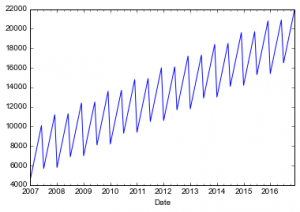The code to generate this plot is:

```import pandas as pd
import statsmodels.api as sm
import matplotlib.pyplot as plt

df.index=pd.to_datetime(df['Date'])
df['Sales'].plot()
plt.show()```

Again it is a good idea to check for stationarity of the time-series. I won’t drag you through the augmented Dickey-Fuller test again, at least right now, since we did it in the last post. We will revisit it to check the stationarity of the residuals from our model. So, for now, just remember that the series looks like it has a unit root, because it does. So we will only consider the first difference.

As a reminder, here are the ACF and PACF plots for the differenced time series. Notice that every sixth ACF component is significant.  Any time you see a regular pattern like that in one of these plots, you should suspect that there is some sort of significant seasonal thing going on. Again, like I showed you in the last post, the idea is to get this thing to be stationary, and you can do that with a complicated error structure, or you can bake the seasonality right in.

Here is the code to reproduce this figure:

```fig = plt.figure(figsize=(12,8))
fig = sm.graphics.tsa.plot_acf(df['Sales'].diff().dropna(), lags=40, ax=ax1)
fig = sm.graphics.tsa.plot_pacf(df['Sales'].diff().dropna(), lags=40, ax=ax2)
plt.show()```

At this point let’s try a SARIMA model with 1 additive MA(6) term, just because that seems to be what the ACF plot is telling us to do, even though we already know the correct model. The code to do this is:

```model=sm.tsa.statespace.SARIMAX(endog=df['Sales'],order=(0,1,0),seasonal_order=(0,0,1,6),trend='c',enforce_invertibility=False)
results=model.fit()
print(results.summary())```

Which will produce this output:

```                                 Statespace Model Results
=========================================================================================
Dep. Variable:                             Sales   No. Observations:                  120
Model:             SARIMAX(0, 1, 0)x(0, 0, 1, 6)   Log Likelihood               -2698.188
Date:                           Fri, 16 Jun 2017   AIC                           5402.376
Time:                                   07:32:09   BIC                           5410.738
Sample:                               01-01-2007   HQIC                          5405.772
- 12-01-2016
Covariance Type:                             opg
==============================================================================
coef    std err          z      P>|z|      [0.025      0.975]
------------------------------------------------------------------------------
intercept    146.2185        nan        nan        nan         nan         nan
ma.S.L6      5.48e+13          0        inf      0.000    5.48e+13    5.48e+13
sigma2      2.621e-09   5.29e-10      4.960      0.000    1.59e-09    3.66e-09
===================================================================================
Ljung-Box (Q):                      715.62   Jarque-Bera (JB):                84.34
Prob(Q):                              0.00   Prob(JB):                         0.00
Heteroskedasticity (H):               1.00   Skew:                            -1.89
Prob(H) (two-sided):                  1.00   Kurtosis:                         4.64
===================================================================================

Warnings:
 Covariance matrix calculated using the outer product of gradients (complex-step).
 Covariance matrix is singular or near-singular, with condition number    inf. Standard errors may be unstable.```

What you will notice is the warnings that come along with this output, once again we have a singular covariance matrix. This is because of the deterministic way that I generated this output. But it still isn’t correct. Notice that I also had to disable the warnings for a matrix that isn’t invertible in order to even get this thing to run. In general, that is a bad idea. I just did it to get some results.

Now for the meat, a genuine perfect fit. A SARIMA(0,1,0)(0,1,0,12) model. The only problem is of course that there is literally nothing to estimate, and so statsmodels is going to yell at us. And it is going to throw out our results.

So since there is just differencing, which you don’t need to run a SARIMA at all to get at the best possible model. To prove it I will provide a one liner of code that will give you residuals of exactly zero for every time period. Here it is:

```print(df['Sales'].diff().diff(12))
```

This one line of code says take the first difference, and then the seasonal difference, and print that out. This gives you residuals of zero for every observation. Unfortunately, that is not very satisfying. By the way, if you want to seasonally adjust this time series, just do this:

```print(df['Sales'].diff(12))
```

That will give you a seasonally adjusted growth rate of \$1200. The first difference bit controls for this growth rate, and you end up with zero. Again, meh. So let’s kick it up a notch with a new dataset. One that is very similar, but has some noise and that noise isn’t just white noise. Here is the code to generate an AR(1) process, with noise having a \$500 standard deviation:

```np.random.seed(5968)
noise=[np.random.normal(scale=500)]

for i in range(len(df)-1):
noise.append(np.random.normal(scale=500)+noise[i]*(-0.85))
df['Sales2']=df['Sales']+noise
df['Sales2'].plot()
plt.show()
```

If you run this code, you will get this output: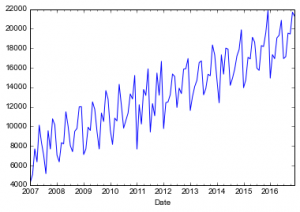What you will notice is that it looks like our sales data from before, but it is much more wiggly and it might even look more “real” to you. And it does. It has some extra noise, which is realistic. For those of you keeping score, this dataset has an AR(1) process with a coefficient on the AR(1) term of -0.85.

I’m going to cheat a little bit, but since we already know that I need a seasonal difference and a total difference, we’ll go ahead and do that, and then we’ll plot the autocorrelations of the differenced series. That is we are plotting the autocorrelations of the residuals of the SARIMA(0,1,0)(0,1,0,12) process. We can do that with this code:

```fig = plt.figure(figsize=(12,8))
fig = sm.graphics.tsa.plot_acf(df['Sales2'].diff().diff(12).dropna(), lags=40, ax=ax1)
fig = sm.graphics.tsa.plot_pacf(df['Sales2'].diff().diff(12).dropna(), lags=40, ax=ax2)
plt.show()

```

That gives you this plot: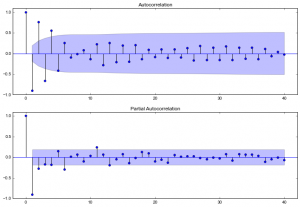This pattern is typical of an AR(1) process with a coefficient of -0.85. Which isn’t unexpected given that we generated the series a few steps back. We also tested for the stationarity of the series, and clearly reject the null of a unit root in favor of a stationary series (Test stat=-4.45 with 1% critical value of -3.50). So we’ll run a SARIMA(1,1,0)(0,1,0,12) model. This will execute, because we have a parameter to estimate.

```model=sm.tsa.statespace.SARIMAX(endog=df['Sales2'],order=(1,1,0),seasonal_order=(0,1,0,12),trend='c',enforce_invertibility=False)
results=model.fit()
print(results.summary())
```

Which gives the following results:

```                                 Statespace Model Results
==========================================================================================
Dep. Variable:                             Sales2   No. Observations:                  120
Model:             SARIMAX(1, 1, 0)x(0, 1, 0, 12)   Log Likelihood                -886.252
Date:                            Mon, 19 Jun 2017   AIC                           1778.505
Time:                                    07:52:06   BIC                           1786.867
Sample:                                01-01-2007   HQIC                          1781.901
- 12-01-2016
Covariance Type:                              opg
==============================================================================
coef    std err          z      P>|z|      [0.025      0.975]
------------------------------------------------------------------------------
intercept    -49.4753     85.956     -0.576      0.565    -217.946     118.995
ar.L1         -0.8991      0.037    -24.005      0.000      -0.973      -0.826
sigma2      8.115e+05   1.13e+05      7.159      0.000    5.89e+05    1.03e+06
===================================================================================
Ljung-Box (Q):                      109.02   Jarque-Bera (JB):                15.01
Prob(Q):                              0.00   Prob(JB):                         0.00
Heteroskedasticity (H):               0.50   Skew:                            -0.47
Prob(H) (two-sided):                  0.04   Kurtosis:                         4.57
===================================================================================

Warnings:
 Covariance matrix calculated using the outer product of gradients (complex-step).
```

Let’s go through this output really fast. The log-likelihood of this regression is just -886.25 which is much lower (in absolute value) than the previous regression that we did in this post which had a log-likelihood of -2698.18. That means that this regression is a better fit of the data. It better be, because we new what the correct model was all along. The intercept in this regression was -\$49.57, but it wasn’t significant. That makes sense since the intercept should have been 0, since the mean of the double differenced series is zero, and without the noise it is exactly zero. The AR(1) term  has a coefficient of -0.8991, with a 95% confidence interval of [-0.826,-0.973], which easily contains the true value of  -0.85. So I’m going to call that a win. Sigma-squared is an estimate of the variability of the residuals, we need it to do the maximum likelihood estimation. Recall that the true noise has a standard deviation of \$500.00, this translates to a sigma-squared of 250,000, or 2.5e5 notice that our estimate was a little high here, but that mainly affects the standard errors, and the rest of our estimates were on point.

Ljung-Box indicates whether or not we can reject the null of all of the autocorrelations being equal to zero in the residuals. We have controlled for the true autocorrelation, so it is a surprise that we reject. The heteroskedasticity test tests for heteroskedasticity (go figure), which is a bit of a surprise, except that our sigma-squared was off. If we used a heteroskedastic robust estimate of the covariance matrix, maybe we would correct for that weird result. The Jarque-Bera test looks for nomality of the residuals by looking at their skew and kurtosis. It is a joint hypothesis test. Again I am surprised to see that it rejects normality. If we look at the skewness, we would have expected an estimate of zero, which is what we see basically at -0.47. And we want a kurtosis of 3, but we got 4.57. So it rejected, probably because of our weird kurtosis. That may be due to the sample that we got. I would play around with the seed that we used to generate the noise. I suspect that the weird results we got will go away by taking a different sample of noise.

Let’s look at what the autocorrelations look like for these residuals: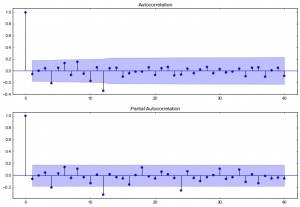Hmmm, it looks like we have a seasonal MA term, the MA(12) term looks significant based on the plot above. This may be due to the fact that we did the seasonal difference before we did the estimation. We may have over differenced the series unintentionally, giving us weird results we were seeing. Let’s try to get back to stationarity by adding that MA(12) term back in.

Let’s try running the numbers again to see what is going on this time we’ll include a seasonal MA term, just to see what happens. Here’s the code.

```model2=sm.tsa.statespace.SARIMAX(endog=df['Sales2'],order=(1,1,0),seasonal_order=(0,1,1,12),trend='c',enforce_invertibility=False)
results2=model2.fit()
print(results2.summary())
```

And here are the results that you get:

```                                 Statespace Model Results
==========================================================================================
Dep. Variable:                             Sales2   No. Observations:                  120
Model:             SARIMAX(1, 1, 0)x(0, 1, 1, 12)   Log Likelihood                -861.879
Date:                            Tue, 20 Jun 2017   AIC                           1731.758
Time:                                    07:23:12   BIC                           1742.908
Sample:                                01-01-2007   HQIC                          1736.286
- 12-01-2016
Covariance Type:                              opg
==============================================================================
coef    std err          z      P>|z|      [0.025      0.975]
------------------------------------------------------------------------------
intercept    -16.0390     22.420     -0.715      0.474     -59.981      27.903
ar.L1         -0.9363      0.028    -33.875      0.000      -0.990      -0.882
ma.S.L12      -0.7668      0.122     -6.285      0.000      -1.006      -0.528
sigma2      4.115e+05   6.41e+04      6.424      0.000    2.86e+05    5.37e+05
===================================================================================
Ljung-Box (Q):                       77.52   Jarque-Bera (JB):                 3.41
Prob(Q):                              0.00   Prob(JB):                         0.18
Heteroskedasticity (H):               0.73   Skew:                            -0.44
Prob(H) (two-sided):                  0.34   Kurtosis:                         3.02
===================================================================================

Warnings:
 Covariance matrix calculated using the outer product of gradients (complex-step).
```

The intercept is still non-significant, the AR term is a little high. The MA term, is looking okay, I guess, it looks like it will absorb some of that over differencing. Sigma-squared is looking much better though. The true value is just outside of the confidence interval.

Ljung-Box suggests that there might be more work to do here. But I think you get the idea so we won’t keep searching for this stationarity. We no longer have heteroskedacticity problems and the residuals appear to be normally distributed. I am, at the risk of being proven wrong, going to declare victory over the noise. It appears that I may have over differenced the time series, by taking the difference and the seasonal difference. That Ljung-Box test statistic does seem to imply that I could do a little bit better, maybe get sigma-squared in the right range, but that is another day, perhaps.

Last thing, here is some code to visually inspect the residuals against the true noise:

```df['noise']=[noise[i]+0.85*noise[i-1] if i>0 else 0 for i in range(len(noise))]
results.resid2.loc['2008-02-01':].plot(label='Regression Residuals')
df['noise'].loc['2008-02-01':].plot(color='r',label='True Noise')
plt.legend(loc=2)
plt.show()
```

Notice how the residuals look a heck of a lot like our noise, but it does seem to indicate that we have slightly larger fluctuations than the true value, that’s probably what the Ljung-Box test is indicating.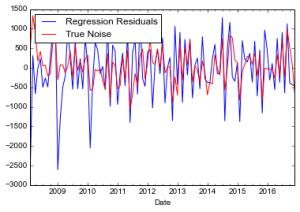As always, here is the full code to reproduce everything, or you can get it from my github repo:

```import pandas as pd
import statsmodels.api as sm
import matplotlib.pyplot as plt
import numpy as np

df.index=pd.to_datetime(df['Date'])
df['Sales'].plot()
plt.show()

fig = plt.figure(figsize=(12,8))
fig = sm.graphics.tsa.plot_acf(df['Sales'].diff().dropna(), lags=40, ax=ax1)
fig = sm.graphics.tsa.plot_pacf(df['Sales'].diff().dropna(), lags=40, ax=ax2)
plt.show()

#This model is shown but not run because it will return an error.
#model=sm.tsa.statespace.SARIMAX(endog=df['Sales'],order=(0,1,0),seasonal_order=(0,1,0,12),trend='c',enforce_invertibility=False)
#results=model.fit()
#print(results.summary())

#To show you why it will return an error use this code:
print(df['Sales'].diff().diff(12))
#%%
np.random.seed(5967)
noise=[np.random.normal(scale=500)]

for i in range(len(df)-1):
noise.append(np.random.normal(scale=500)+noise[i]*(-0.85))
df['Sales2']=df['Sales']+noise
df['Sales2'].plot()
plt.show()

#%%
fig = plt.figure(figsize=(12,8))
fig = sm.graphics.tsa.plot_acf(df['Sales2'].diff().diff(12).dropna(), lags=40, ax=ax1)
fig = sm.graphics.tsa.plot_pacf(df['Sales2'].diff().diff(12).dropna(), lags=40, ax=ax2)
plt.show()

model=sm.tsa.statespace.SARIMAX(endog=df['Sales2'],order=(1,1,0),seasonal_order=(0,1,0,12),trend='c',enforce_invertibility=False)
results=model.fit()
print(results.summary())
#%%
fig = plt.figure(figsize=(12,8))
fig = sm.graphics.tsa.plot_acf(results.resid, lags=40, ax=ax1)
fig = sm.graphics.tsa.plot_pacf(results.resid, lags=40, ax=ax2)
plt.show()

df['noise']=noise
results.resid.loc['2008-02-01':].plot(label='Regression Residuals')
df['noise'].loc['2008-02-01':].plot(color='r',label='True Noise')
plt.legend(loc=2)
plt.show()

#%%
model2=sm.tsa.statespace.SARIMAX(endog=df['Sales2'],order=(1,1,0),seasonal_order=(0,1,1,12),trend='c',enforce_invertibility=False)
results2=model2.fit()
print(results2.summary())

fig = plt.figure(figsize=(12,8))
fig = sm.graphics.tsa.plot_acf(results2.resid, lags=40, ax=ax1)
fig = sm.graphics.tsa.plot_pacf(results2.resid, lags=40, ax=ax2)
plt.show()

df['noise']=[noise[i]+0.85*noise[i-1] if i>0 else 0 for i in range(len(noise))]
results2.resid.loc['2008-02-01':].plot(label='Regression Residuals')
df['noise'].loc['2008-02-01':].plot(color='r',label='True Noise')
plt.legend(loc=2)
plt.show()
```
Published

1.Simon says:

hi. I will admit I have just skim read the article on the way home from work. I intend to read it properly on Monday.

However what’s not clear to me is can I have multiple seasonal periods. e.g I have hourly data, where there is daily weekly and yearly periodicly. is it possible to implement all three at once?

Cheers. and apologies if this is already mentioned

1.Ryan Barnes says:

Hey Simon,

Thanks for reaching out. I hope you enjoy the read!

That is a good question, the short answer is yes you can do this. But the way you implement it would be very, very annoying. It would involve making a vector like I did in the post above to record whether or not there is a seasonal element. However, to implement a daily periodicity you would need a vector/array of 24 elements, where the last one was a 1. To get daily and weekly periodicity you would need to extend the array to be 168 elements long where all of the elements are zero except for the 24th and 168th element. And if you want all three you need an array that is 8760 elements long with elements 24,168, and 8760 set to 1 zeroes everywhere else. I’m thinking something along the lines of this to build the array:

```periods =  * 8760
periods = 1
periods = 1
periods = 1
```

Then you just need to plug this array into the call to the SARIMA model. I hope that helps, good luck and let me know how it goes.

2.Lalitha Raghavan says:

Hi Thanks for this useful post. I am still a newbie so my questions may seem too basic, but I want to understand the this.

An ARIMA model can be employed only if there is stationarity. I think I have read this in multiple places. Now, does the same apply for SARIMA as well? Do I need to remove the trend and seasonality in my data in order to process it in SARIMA?

1.Ryan Barnes says:

Yes that is the basic idea you need to remove seasonality, trend, AR, and MA to get a stationary serieson which to do your regression.

That is what SARIMA does. It gets your series to that point so that you are dealing with a stationary series.

1.Lalitha Raghavan says:

So just to clarify once again, SARIMA does this for me or do I have to clean the data for seasonality and trends before submitting to SARIMA?

3.Sumit Sengupta says:

Hi Ryan,

4.Sanket says:
1.Ryan Barnes says: